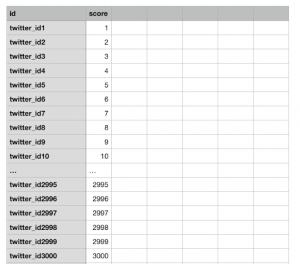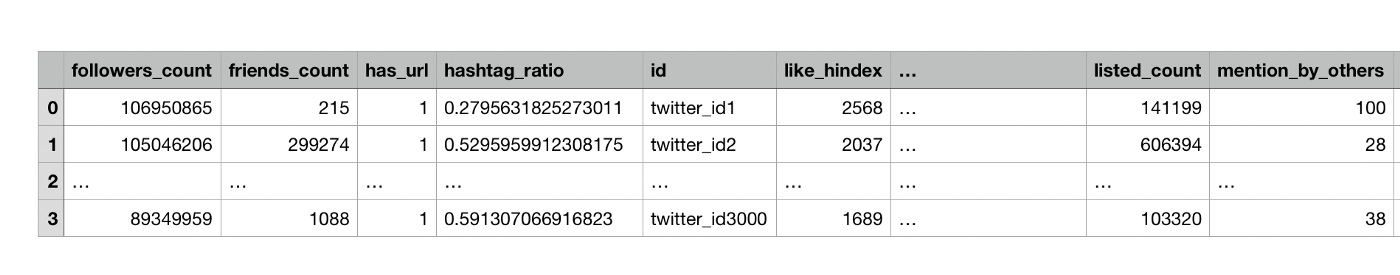Let's Discuss

Enquire Now

# Build an Influencer Score Model using Linear Regression

Jun 7, 2022by, Mythili S

Machine Learning

An influencer is the one who can make an impact on a service/product by giving his opinion towards it. Thereby he can influence his friends, peers or his followers on platforms such as Twitter, Facebook, Instagram, etc. They can also associate with popular brands across the globe and promote their products.

So what about predicting the influence of any user for a particular platform?

Interesting.. right?

In this blog post we will build such a model and predict the influencer score of those users.

Let’s do it for the Twitter platform.

• Deciding the Features : Features that are to be extracted for building the model.

For e.g., followers_count, friends_count, listed_count etc.

• Collecting Twitter IDs: Twitter IDs of users are to be fetched (all kinds of users, including influencer and normal users). These are to be ranked according to our criteria.
• Feature extraction: Extracting the features of collected users.
• Building the model: Building a linear regression model using the extracted features.
• Prediction :  Predicting the influencer score of a twitter user using the model.

## Deciding the Features

An Influencer must have certain characteristics such as stimulating a discussion, proposing ideas, following up activities, etc. These characteristics can translate to its equivalent numeric representation using the user’s meta information and the content published by the user. So, the parameters which affect the influencer score of users can be identified. It may vary according to the platform since the metadata availability depends on the platform.

For any given Twitter user, we can collect and compute the following features associated with the user.

• total followers: (followers_count)
• total following: (friends_count)
• total listings: (listed_count)
• total favourites: (favourites_count)
• total tweets by the user: (statuses count)
• hasURL: Denotes if URL is present in profile (true or false)
• mention_by_others: Number of times that user is mentioned in tweets by others
• retweet_ratio: Number of times that user’s tweet is retweeted / Total original tweets in DB
• liked_ratio: Number of times that user’s tweet is liked by other Twitter users
• orig_content_ratio: (Total tweets in DB – retweets by that user ) / Total tweets in DB
• hashtag_ratio: Total tweets by that user that contain one or more HASHTAGS / Total tweets in DB
• urls_ratio: Total tweets by that user that contain one or more URLs / Total tweets in DB
• symbols_ratio: Total tweets by that user that contain one or more SYMBOLS / Total tweets in DB
• mentions_ratio: Total tweets by that user that contain one or more @mentions / Total tweets in DB
• reputation: Social score that depends only on followers, following and number of tweets.
• retweet_hindex: (similar to citation [h-index][hindex link])
• like_hindex: (similar to citation [h-index][hindex link])

In order to extract posts and features of a user, we need to have that user’s twitter id. For this article, nearly 2000 twitter ids of normal users and 1000 influencers are collected from here. After collecting these ids, ranks are to be assigned manually (by giving score) to these users.## Feature Extraction

From the collected twitter ids, the features of each tweet of the user are to be extracted. For that, the python library tweepy (https://github.com/tweepy/tweepy) is used.

It can be installed by:

```
pip install tweepy ```

In order to use tweepy we need to have tokens associated with a twitter developer account. For creating a twitter developer account, follow this link.

```import tweepy
import sys, os, csv

##use tokens from dev account
CONSUMER_KEY = 'consumer_key'
CONSUMER_SECRET = 'consumer_secret'
OAUTH_TOKEN = 'oath_token'
OAUTH_TOKEN_SECRET = 'oath_token_secret'

auth = tweepy.OAuthHandler(CONSUMER_KEY, CONSUMER_SECRET)
auth.secure = True
auth.set_access_token(OAUTH_TOKEN, OAUTH_TOKEN_SECRET)
tweepyApi = tweepy.API(auth, wait_on_rate_limit=True,
wait_on_rate_limit_notify=True,
parser=tweepy.parsers.JSONParser())```

It will take several hours to extract all those features as twitter APIs are rate limited and finally we will get a CSV file containing all the extracted features.

##Building the Model

Let’s take a look at the correlation of each feature with the influence score. Most of the features have some correlation with influence score, which means there is no need to drop any feature.

```corr = features.corr()
print("Correlation of features with Influence score \n")
print corr['score']```

To learn and evaluate a linear regression model for influence score, we create a training and test set by splitting the dataset by a 3:2 ratio.

``` def make_train_test_set(df, train_test_split_prct,
clipping_qunatile):
msk = np.random.rand(len(df)) < train_test_split_prct
train_df = df[msk].copy()
test_df = df[~msk].copy()
thres  = train_df.quantile(clipping_qunatile)
fet_list = [ x for x in list(df) if x not in ['id',
'screen_name'] ]
for col in fet_list:
if col :
train_df[col] = train_df[col]/thres[col]
test_df[col] = test_df[col]/thres[col]
train_df.clip(0, 1)
test_df.clip(0,1)
cols = [col for col in list(df) if col not in ["score",
'screen_name', 'id']]
y_train = train_df['score'].values
y_test = test_df['score'].values
X_train = train_df[cols].values
X_test = test_df[cols].values
return X_train, X_test, y_train, y_test, thres.transpose(),
fet_list```

Also, most of the features have no upper bound and hence can have outlier values. Let’s use the 95th-percentile value of each feature value to clip the feature and use min-max normalization to normalize the features to 0-1 range.

```import json
def get_mean_clip_value():
thres_list = []
for run in range(1, 50):
X_train, X_test, y_train, y_test, thres, fet_list =
make_train_test_set()
thres_list.append(thres)
thres_df = pd.DataFrame(thres_list)
return thres_df.mean()
def LinearRegressionModel():
X_train, X_test, y_train, y_test, thres, fet_clip_value =
make_train_test_set()
regr = linear_model.LinearRegression()
regr.fit(X_train, y_train)
return regr, fet_list, fet_clip_value
def SerializeLinearRegressionModel(model_path):
model, fet_list, clip_value = LinearRegressionModel()
clip_value = get_mean_clip_value()
jsonObj = {}
modelDict = {}
normalizationDict = {}
coeffDict = dict(zip(fet_list, list(model.coef_)))
modelDict['coeff'] = coeffDict
modelDict['intercept'] = model.intercept_
for fet in fet_list:
normalizationDict[fet] = clip_value[fet]
jsonObj['LRModel'] = modelDict
jsonObj['clippingValue'] = normalizationDict
jsonStr = json.dumps(jsonObj, indent=4, sort_keys=True)
with open(model_path, 'wb') as f:
f.write(jsonStr)

def DeserializeLinearRegressionModel(model_path):
with open(model_path) as f:
return model
blob_service.get_blob_to_path(mycontainer,
DeserializeLinearRegressionModel(model_path)```

## Prediction

For any new twitter user, a similar process is followed to predict the influence score.

• Extract several tweets of the user using tweepy
• Normalize the feature
• Predict the influence score using the model.
```def normalize_features(fet_vec, model):
normalize_fet = {}
clip_dict = model["clippingValue"]
for fet in fet_vec.keys():
if fet in clip_dict.keys():
normalize_fet[fet] = max(0, min(1,
float(fet_vec[fet])/float(clip_dict[fet])))
return normalize_fet
def compute_influence_score(feature_vec, model):
score = 0
coeff_dict = model["LRModel"]["coeff"]
intercept = float(model["LRModel"]["intercept"])
for fet in feature_vec.keys():
if fet in coeff_dict.keys():
score = score + float(feature_vec[fet]) *
float(coeff_dict[fet])
score += intercept
return max(min(1, score), 0)
tweets, mentions = get_all_tweets(screen_name)
features = compute_features(tweets, mentions)
normalize_feature = normalize_features(features,
predictionModel)
influence_score =
compute_influence_score(normalize_feature, predictionModel)
print ("\nInfluence Score of {0}:
{1:.3f}\n".format(screen_name, influence_score))```

Provide one or more usernames to compute their influence score,

```twitter_user_names = ['imVkohli', 'StephenCurry30']